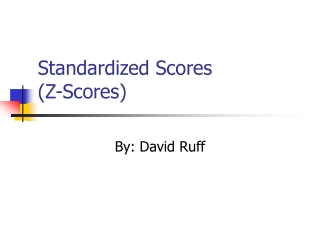DownloadDownload PresentationStandardized Scores (Z-Scores)

# Standardized Scores (Z-Scores)

Télécharger la présentation## Standardized Scores (Z-Scores)

- - - - - - - - - - - - - - - - - - - - - - - - - - - E N D - - - - - - - - - - - - - - - - - - - - - - - - - - -
##### Presentation Transcript

1. Standardized Scores(Z-Scores) By: David Ruff

2. Z-Score Defined • The number of standard deviations a raw score (individual score) deviates from the mean

3. X - X sx where: Zx= standardized score for a value of X = number of standard deviations a raw score (X-score) deviates from the mean X= an interval/ratio variable X= the mean of X sx= the standard deviation of X Computing Z-Score ¯ Zx = ¯

4. Direction of a Z-score • The sign of any Z-score indicates the direction of a score: whether that observation fell above the mean (the positive direction) or below the mean (the negative direction) • If a raw score is below the mean, the z-score will be negative, and vice versa

5. Comparing variables with very different observed units of measure • Example of comparing an SAT score to an ACT score • Mary’s ACT score is 26. Jason’s SAT score is 900. Who did better? • The mean SAT score is 1000 with a standard deviation of 100 SAT points. The mean ACT score is 22 with a standard deviation of 2 ACT points.

6. Jason: 900-1000 100 Mary: 26-22 2 From these findings, we gather that Jason’s score is 1 standard deviation below the mean SAT score and Mary’s score is 2 standard deviations above the mean ACT score. Therefore, Mary’s score is relatively better. Let’s find the z-scores Zx = = -1 = Zx = +2

7. Z-scores and the normal curve SD SD SD SD SD SD SD SD SD 68% 95% 99%

8. Interpreting the graph • For any normally distributed variable: • 50% of the scores fall above the mean and 50% fall below. • Approximately 68% of the scores fall within plus and minus 1 Z-score from the mean. • Approximately 95% of the scores fall within plus and minus 2 Z-scores from the mean. • 99.7% of the scores fall within plus and minus 3 Z-scores from the mean.

9. Example • Suppose a student is applying to various law schools and wishes to gain an idea of what his GPA and LSAT scores will need to be in order to be admitted. • Assume the scores are normally distributed • The mean GPA is a 3.0 with a standard deviation of .2 • The mean LSAT score is a 155 with a standard deviation of 7

10. GPA SD SD SD SD SD SD SD 2.4 2.6 2.8 3.0 3.2 3.4 3.6 68% 95% 99%

11. LSAT Scores SD SD SD SD SD SD SD 134 141 148 155 162 169 176 68% 95% 99%

12. What we’ve learned • The more positive a z-score is, the more competitive the applicant’s scores are. • The top 16% for GPA is from a 3.2 upwards; for LSAT score, from 162 upwards. • The top 2.5% for GPA from a 3.2 upwards; for LSAT score, from 169 upwards. • An LSAT score of 176 falls within the top 1%, as does a GPA of 3.6. • Lesson: the z-score is a great tool for analyzing the range within which a certain percentage of a population’s scores will fall.

13. Conclusions • Z-score is defined as the number of standard deviations from the mean. • Z-score is useful in comparing variables with very different observed units of measure. • Z-score allows for precise predictions to be made of how many of a population’s scores fall within a score range in a normal distribution.

14. Works Cited • Ritchey, Ferris. The Statistical Imagination. New York: McGraw- Hill, 2000. • Tushar Mehta Excel Page. <http://www.tushar- mehta.com/excel/charts/normal_dist ribution/>